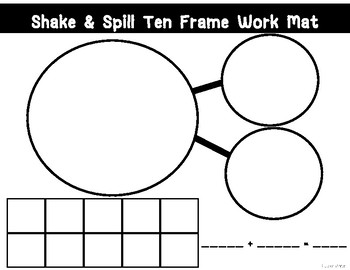# Shake & Spill Ten Frame Work MatSubject
Resource Type
File Type
PDF (235 KB|2 pages)
Standards
\$1.50
Also included in:
1. 45% off on all of my work mats when you purchase this bundle!Bonus Work Mats INCLUDED! (10 pages no where else in my store)Can be used with whole group, small group, or centers. Made to be easily differentiated, and student-led. Print on colored card-stock, laminate or place in a clear plastic sleev
\$27.00
\$15.00
Save \$12.00
• Product Description
• Standards

Use in whole group, small group, or in centers.

Print on color card-stock, laminate or place inside a clear plastic sleeve. Students use dry-erase markers.

Have students use counters, shake and spill, sort counters by color, fill in ten frame and number sentence.

Look for and express regularity in repeated reasoning. Mathematically proficient students notice if calculations are repeated, and look both for general methods and for shortcuts. Upper elementary students might notice when dividing 25 by 11 that they are repeating the same calculations over and over again, and conclude they have a repeating decimal. By paying attention to the calculation of slope as they repeatedly check whether points are on the line through (1, 2) with slope 3, middle school students might abstract the equation (𝑦 – 2)/(𝑥 – 1) = 3. Noticing the regularity in the way terms cancel when expanding (𝑥 – 1)(𝑥 + 1), (𝑥 – 1)(𝑥² + 𝑥 + 1), and (𝑥 – 1)(𝑥³ + 𝑥² + 𝑥 + 1) might lead them to the general formula for the sum of a geometric series. As they work to solve a problem, mathematically proficient students maintain oversight of the process, while attending to the details. They continually evaluate the reasonableness of their intermediate results.
Look for and make use of structure. Mathematically proficient students look closely to discern a pattern or structure. Young students, for example, might notice that three and seven more is the same amount as seven and three more, or they may sort a collection of shapes according to how many sides the shapes have. Later, students will see 7 × 8 equals the well remembered 7 × 5 + 7 × 3, in preparation for learning about the distributive property. In the expression 𝑥² + 9𝑥 + 14, older students can see the 14 as 2 × 7 and the 9 as 2 + 7. They recognize the significance of an existing line in a geometric figure and can use the strategy of drawing an auxiliary line for solving problems. They also can step back for an overview and shift perspective. They can see complicated things, such as some algebraic expressions, as single objects or as being composed of several objects. For example, they can see 5 – 3(𝑥 – 𝑦)² as 5 minus a positive number times a square and use that to realize that its value cannot be more than 5 for any real numbers 𝑥 and 𝑦.
Use appropriate tools strategically. Mathematically proficient students consider the available tools when solving a mathematical problem. These tools might include pencil and paper, concrete models, a ruler, a protractor, a calculator, a spreadsheet, a computer algebra system, a statistical package, or dynamic geometry software. Proficient students are sufficiently familiar with tools appropriate for their grade or course to make sound decisions about when each of these tools might be helpful, recognizing both the insight to be gained and their limitations. For example, mathematically proficient high school students analyze graphs of functions and solutions generated using a graphing calculator. They detect possible errors by strategically using estimation and other mathematical knowledge. When making mathematical models, they know that technology can enable them to visualize the results of varying assumptions, explore consequences, and compare predictions with data. Mathematically proficient students at various grade levels are able to identify relevant external mathematical resources, such as digital content located on a website, and use them to pose or solve problems. They are able to use technological tools to explore and deepen their understanding of concepts.
Determine the unknown whole number in an addition or subtraction equation relating three whole numbers. For example, determine the unknown number that makes the equation true in each of the equations 8 + ? = 11, 5 = ▯ - 3, 6 + 6 = ▯.
Understand the meaning of the equal sign, and determine if equations involving addition and subtraction are true or false. For example, which of the following equations are true and which are false? 6 = 6, 7 = 8 - 1, 5 + 2 = 2 + 5, 4 + 1 = 5 + 2.
Total Pages
2 pages